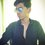# This cannot happen

Today I was going to post a problem but i suddenly got confused about problem.The problem was if $$\sqrt { \sqrt { 14-\sqrt { 132 } } +\sqrt { 23-\sqrt { 528 } + } \sqrt { 3 } } =a$$.Find the value of $$a$$.

My solution is following:

$\sqrt { 14-\sqrt { 132 }}=3+11-2\sqrt { 132 }=\sqrt { { \left( \sqrt { 3 } -\sqrt { 11 } \right) }^{ 2 } }$

Similarly

$\sqrt { 23-\sqrt { 528 } }=\sqrt { { \left( \sqrt { 11 } -\sqrt { 12 } \right) }^{ 2 } }$

And $\sqrt { 3 }=\sqrt {15-12}=\sqrt { 12+3-6\times 2}=\sqrt { \sqrt { { 12 }^{ 2 } } +\sqrt { { 3 }^{ 2 } } -2\sqrt { 36 }} =\sqrt { { \left( \sqrt { 12 } -\sqrt { 3 } \right) }^{ 2 } }$

Therefore

$\sqrt { \sqrt { 14-\sqrt { 132 } } +\sqrt { 23-\sqrt { 528 } + } \sqrt { 3 } } =\sqrt { { \left( \sqrt { 3 } -\sqrt { 11 } \right) }^{ 2 } } +\sqrt { { \left( \sqrt { 11 } -\sqrt { 12 } \right) }^{ 2 } } +\sqrt { { \left( \sqrt { 12 } -\sqrt { 3 } \right) }^{ 2 } } =0$

But now use calculator and evaluate i get answer above 0.

Please help me about this.Which step did I do wrong.Note by Shivamani Patil
6 years, 9 months ago

This discussion board is a place to discuss our Daily Challenges and the math and science related to those challenges. Explanations are more than just a solution — they should explain the steps and thinking strategies that you used to obtain the solution. Comments should further the discussion of math and science.

When posting on Brilliant:

• Use the emojis to react to an explanation, whether you're congratulating a job well done , or just really confused .
• Ask specific questions about the challenge or the steps in somebody's explanation. Well-posed questions can add a lot to the discussion, but posting "I don't understand!" doesn't help anyone.
• Try to contribute something new to the discussion, whether it is an extension, generalization or other idea related to the challenge.
• Stay on topic — we're all here to learn more about math and science, not to hear about your favorite get-rich-quick scheme or current world events.

MarkdownAppears as
*italics* or _italics_ italics
**bold** or __bold__ bold
- bulleted- list
• bulleted
• list
1. numbered2. list
1. numbered
2. list
Note: you must add a full line of space before and after lists for them to show up correctly
paragraph 1paragraph 2

paragraph 1

paragraph 2

[example link](https://brilliant.org)example link
> This is a quote
This is a quote
    # I indented these lines
# 4 spaces, and now they show
# up as a code block.

print "hello world"
# I indented these lines
# 4 spaces, and now they show
# up as a code block.

print "hello world"
MathAppears as
Remember to wrap math in $$ ... $$ or $ ... $ to ensure proper formatting.
2 \times 3 $2 \times 3$
2^{34} $2^{34}$
a_{i-1} $a_{i-1}$
\frac{2}{3} $\frac{2}{3}$
\sqrt{2} $\sqrt{2}$
\sum_{i=1}^3 $\sum_{i=1}^3$
\sin \theta $\sin \theta$
\boxed{123} $\boxed{123}$

## Comments

Sort by:

Top Newest

Convention is that $\sqrt { x }$ returns a positive value, even though it can be both positive and negative. Hence, if we rewrite your expression in this way

$\sqrt { { (\sqrt { 11 } -\sqrt { 3 } ) }^{ 2 } } +\sqrt { { (\sqrt { 12 } -\sqrt { 11 } ) }^{ 2 } } +\sqrt { { (\sqrt { 12 } -\sqrt { 3 } ) }^{ 2 } }$

you'll get a non-zero value which agrees with the original expression.

This is one of the big problems with the idea that a "function can only return one value". By insisting that functions can "only return one value", we're missing out the big picture. It's an artificial restriction. I prefer using implicit equations for exactly that reason, it takes care of all the problems with signs. For example, $y=\sqrt { 1-{ x }^{ 2 } }$ is not even a circle, it's only a half of a circle. But ${ y }^{ 2 }=1-{ x }^{ 2 }$ is a circle.

- 6 years, 9 months ago

Log in to reply

How did you get above expression?

- 6 years, 9 months ago

Log in to reply

It's exactly the same as yours, except that I reversed the order of some of the terms, which I can do since, after being squared, it's the same. That is, for example, in the 2nd line, you could have reversed the terms of the expression on the far right with equal mathematical validity.

- 6 years, 9 months ago

Log in to reply

If i want to post this problem then what conditions i should mention to make it valid problem.

- 6 years, 8 months ago

Log in to reply

A calculator will always return a postive square root. Roank above has given a good explanation.

- 6 years, 8 months ago

Log in to reply

The big mistake was $\sqrt{{x}^{2}}=\left| x \right|$ and not $x$. Hope this clarifies things a bit.

- 6 years, 9 months ago

Log in to reply

×

Problem Loading...

Note Loading...

Set Loading...